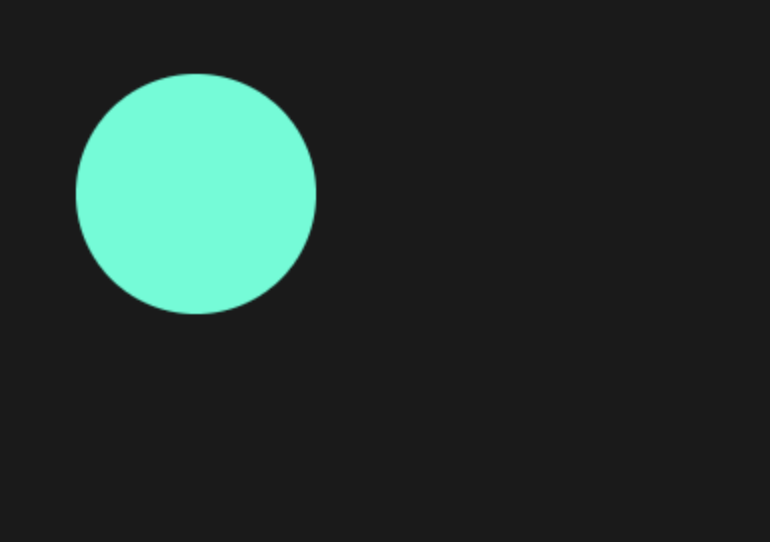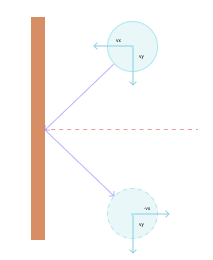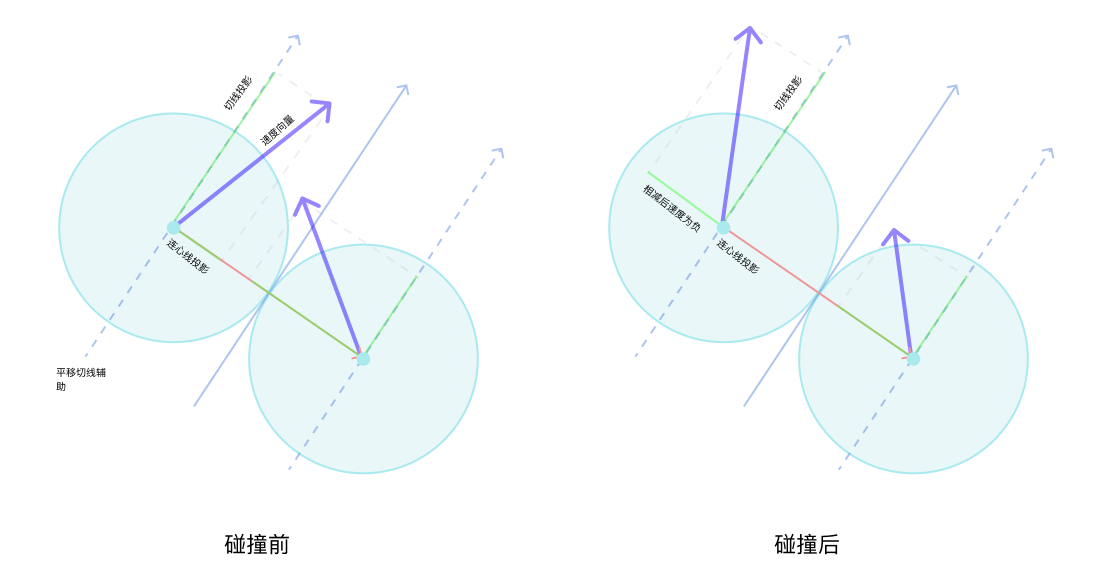# JavaScript 游戏开发 - 物理碰撞引擎实现

## 基础结构​

<link rel="stylesheet" href="./style.css" />

<body /> 中，添加 canvas 元素、加载 index.js 文件：

<main>  <canvas id="gameboard"></canvas></main><script src="./index.js"></script>

style.css 中的代码如下：

* {  box-sizing: border-box;  padding: 0;  margin: 0;  font-family: sans-serif;}main {  width: 100vw;  height: 100vh;  background: hsl(0deg, 0%, 10%);}

hsl(hue, saturation, brightness) 为 css 颜色表示法之一，参数分别为色相，饱和度和亮度，可通过我之前出过的视频进行学习。

## 绘制小球​

const canvas = document.getElementById("gameboard");const ctx = canvas.getContext("2d");canvas.width = window.innerWidth;canvas.height = window.innerHeight;let width = canvas.width;let height = canvas.height;ctx.fillStyle = "hsl(170, 100%, 50%)";ctx.beginPath();ctx.arc(100, 100, 60, 0, 2 * Math.PI);ctx.fill();

• 通过 canvas 的 id 获取 canvas 元素对象。
• 通过 canvas 元素对象获取绘图 context， getContext() 需要一个参数，用于表明是绘制 2d 图像，还是使用 webgl 绘制 3d 图象，这里选择 2d。context 就类似是一支画笔，可以改变它的颜色和绘制基本的形状。
• 给 canvas 的宽高设置为浏览器可视区域的宽高，并保存到 widthheight 变量中方便后续使用。
• 给 context 设置颜色，然后调用 beginPath() 开始绘图。
• 使用 arc() 方法绘制圆形，它接收 5 个参数，前两个为圆心的 x、y 坐标，第 3 个为半径长度， 第 4 个和第 5 个分别是起始角度和结束角度，因为 arc() 其实是用来绘制一段圆弧，这里让它画一段 0 到 360 度的圆弧，就形成了一个圆形。这里的角度是使用 radian 形式表示的，0 到 360 度可以用 0 到 2 * Math.PI 来表示。
• 最后使用 ctx.fill() 给圆形填上颜色。## 移动小球​

Canvas 进行动画的原理与传统的电影胶片类似，在一段时间内，绘制图像、更新图像位置或形状、清除画布，重新绘制图像，当在 1 秒内连续执行 60 次或以上这样的操作时，即以 60 帧的速度，就可以产生连续的画面。

function process() {  window.requestAnimationFrame(process);}window.requestAnimationFrame(process);

let x = 100;let y = 100;let vx = 12;let vy = 25;function process() {  ctx.fillStyle = "hsl(170, 100%, 50%)";  ctx.beginPath();  ctx.arc(x, y, 60, 0, 2 * Math.PI);  ctx.fill();  window.requestAnimationFrame(process);}window.requestAnimationFrame(process);

let startTime;function process(now) {  if (!startTime) {    startTime = now;  }  let seconds = (now - startTime) / 1000;  startTime = now;  // 更新位置  x += vx * seconds;  y += vy * seconds;  // 清除画布  ctx.clearRect(0, 0, width, height);  // 绘制小球  ctx.fillStyle = "hsl(170, 100%, 50%)";  ctx.beginPath();  ctx.arc(x, y, 60, 0, 2 * Math.PI);  ctx.fill();  window.requestAnimationFrame(process);}

process() 现在接收当前时间戳作为参数，然后做了下面这些操作：

• 计算上次函数调用与本次函数调用的时间间隔，以秒计，记录本次调用的时间戳用于下一次计算。
• 根据 x、y 方向上的速度，和刚刚计算出来的时间，计算出移动距离。
• 调用 clearRect() 清除矩形区域画布，这里的参数，前两个是左上角坐标，后两个是宽高，把 canvas 的宽高传进去就会把整个画布清除。
• 重新绘制小球。

## 重构代码​

class Circle {  constructor(context, x, y, r, vx, vy) {    this.context = context;    this.x = x;    this.y = y;    this.r = r;    this.vx = vx;    this.vy = vy;  }      // 绘制小球  draw() {    this.context.fillStyle = "hsl(170, 100%, 50%)";    this.context.beginPath();    this.context.arc(this.x, this.y, this.r, 0, 2 * Math.PI);    this.context.fill();  }  /**   * 更新画布   * @param {number} seconds   */  update(seconds) {    this.x += this.vx * seconds;    this.y += this.vy * seconds;  }}

class Gameboard {  constructor() {    this.startTime;    this.init();  }  init() {    this.circles = [      new Circle(ctx, 100, 100, 60, 12, 25),      new Circle(ctx, 180, 180, 30, 70, 45),    ];    window.requestAnimationFrame(this.process.bind(this));  }  process(now) {    if (!this.startTime) {      this.startTime = now;    }    let seconds = (now - this.startTime) / 1000;    this.startTime = now;    for (let i = 0; i < this.circles.length; i++) {      this.circles[i].update(seconds);    }    ctx.clearRect(0, 0, width, height);    for (let i = 0; i < this.circles.length; i++) {      this.circles[i].draw(ctx);    }    window.requestAnimationFrame(this.process.bind(this));  }}new Gameboard();

• startTime 保存了上次函数执行的时间戳的属性，放到了构造函数中。
• init() 方法创建了一个 circles 数组，里边放了两个示例的小球，这里先不涉及碰撞问题。然后调用 window.requestAnimationFrame() 开启动画。注意这里使用了 bind() 来把 Gameboard 的 this 绑定到回调函数中，以便于访问 Gameboard 中的方法和属性。
• process() 方法也写到了这里边，每次执行时会遍历小球数组，对每个小球进行位置更新，然后清除画布，再重新绘制每个小球。
• 最后初始化 Gameboard 对象就可以开始执行动画了。

## 碰撞检测​

this.circles = [  new Circle(ctx, 30, 50, 30, -100, 390),  new Circle(ctx, 60, 180, 20, 180, -275),  new Circle(ctx, 120, 100, 60, 120, 262),  new Circle(ctx, 150, 180, 10, -130, 138),  new Circle(ctx, 190, 210, 10, 138, -280),  new Circle(ctx, 220, 240, 10, 142, 350),  new Circle(ctx, 100, 260, 10, 135, -460),  new Circle(ctx, 120, 285, 10, -165, 370),  new Circle(ctx, 140, 290, 10, 125, 230),  new Circle(ctx, 160, 380, 10, -175, -180),  new Circle(ctx, 180, 310, 10, 115, 440),  new Circle(ctx, 100, 310, 10, -195, -325),  new Circle(ctx, 60, 150, 10, -138, 420),  new Circle(ctx, 70, 430, 45, 135, -230),  new Circle(ctx, 250, 290, 40, -140, 335),];

class Circle {  constructor(context, x, y, r, vx, vy) {    // 其它代码    this.colliding = false;  }  draw() {    this.context.fillStyle = this.colliding      ? "hsl(300, 100%, 70%)"      : "hsl(170, 100%, 50%)";    // 其它代码  }}

$\sqrt{(x_1 - x_2)^2 + (y_1 - y_2)^2}$

x1、y1 和 x2、y2 分别两个小球的圆心坐标。在比较时，可以对半径和进行平方运算，进而省略对距离的开方运算，也就是可以用下方的公式进行比较：

$(x_1 - x_2)^2 + (y_1 - y_2)^2 \leq (r_1 + r_2)^2$

r1 和 r2 为两球的半径。

isCircleCollided(other) {  let squareDistance =      (this.x - other.x) * (this.x - other.x) +      (this.y - other.y) * (this.y - other.y);  let squareRadius = (this.r + other.r) * (this.r + other.r);  return squareDistance <= squareRadius;}

checkCollideWith(other) {  if (this.isCircleCollided(other)) {    this.colliding = true;    other.colliding = true;  }}

checkCollision() {  // 重置碰撞状态  this.circles.forEach((circle) => (circle.colliding = false));  for (let i = 0; i < this.circles.length; i++) {    for (let j = i + 1; j < this.circles.length; j++) {      this.circles[i].checkCollideWith(this.circles[j]);    }  }}

for (let i = 0; i < this.circles.length; i++) {  this.circles[i].update(seconds);}this.checkCollision();

## 边界碰撞​checkEdgeCollision() {  this.circles.forEach((circle) => {    // 左右墙壁碰撞    if (circle.x < circle.r) {      circle.vx = -circle.vx;      circle.x = circle.r;    } else if (circle.x > width - circle.r) {      circle.vx = -circle.vx;      circle.x = width - circle.r;    }    // 上下墙壁碰撞    if (circle.y < circle.r) {      circle.vy = -circle.vy;      circle.y = circle.r;    } else if (circle.y > height - circle.r) {      circle.vy = -circle.vy;      circle.y = height - circle.r;    }  });}

this.checkEdgeCollision();this.checkCollision();

## 向量的基本操作​

class Vector {  constructor(x, y) {    this.x = x;    this.y = y;  }  /**   * 向量加法   * @param {Vector} v   */  add(v) {    return new Vector(this.x + v.x, this.y + v.y);  }  /**   * 向量减法   * @param {Vector} v   */  substract(v) {    return new Vector(this.x - v.x, this.y - v.y);  }  /**   * 向量与标量乘法   * @param {Vector} s   */  multiply(s) {    return new Vector(this.x * s, this.y * s);  }  /**   * 向量与向量点乘（投影）   * @param {Vector} v   */  dot(v) {    return this.x * v.x + this.y * v.y;  }  /**   * 向量标准化（除去长度）   * @param {number} distance   */  normalize() {    let distance = Math.sqrt(this.x * this.x + this.y * this.y);    return new Vector(this.x / distance, this.y / distance);  }}

## 碰撞处理​##### 动量守恒定律​
$m_1v_1 + m_2v_2 = m_1v_1' + m_2v_2'$
##### 动能守恒定律​
$\frac{1}{2}m_1v_1^2+\frac{1}{2}m_2v_2^2=\frac{1}{2}m_1v_1'^2+\frac{1}{2}m_2v_2'^2$

m1、m2 分别为两小球的质量，v1 和 v2 为两小球碰撞前的速度向量，v1' 和 v2' 为碰撞后的速度向量。根据这两个公式可以推导出两小球碰撞后的速度公式：

$v_1'=\frac{v_1(m_1-m_2)+2m_2v_2}{m_1+m_2}$
$v_2'=\frac{v_2(m_2-m_1)+2m_1v_1}{m_1+m_2}$

$v_1'=v_2$
$v_2'=v_1$

class Circle {  constructor(context, x, y, r, vx, vy, mass = 1) {    // 其它代码    this.mass = mass;  }}

this.circles = [  new Circle(ctx, 30, 50, 30, -100, 390, 30),  new Circle(ctx, 60, 180, 20, 180, -275, 20),  new Circle(ctx, 120, 100, 60, 120, 262, 100),  new Circle(ctx, 150, 180, 10, -130, 138, 10),  new Circle(ctx, 190, 210, 10, 138, -280, 10),  new Circle(ctx, 220, 240, 10, 142, 350, 10),  new Circle(ctx, 100, 260, 10, 135, -460, 10),  new Circle(ctx, 120, 285, 10, -165, 370, 10),  new Circle(ctx, 140, 290, 10, 125, 230, 10),  new Circle(ctx, 160, 380, 10, -175, -180, 10),  new Circle(ctx, 180, 310, 10, 115, 440, 10),  new Circle(ctx, 100, 310, 10, -195, -325, 10),  new Circle(ctx, 60, 150, 10, -138, 420, 10),  new Circle(ctx, 70, 430, 45, 135, -230, 45),  new Circle(ctx, 250, 290, 40, -140, 335, 40),];

  changeVelocityAndDirection(other) {    // 创建两小球的速度向量    let velocity1 = new Vector(this.vx, this.vy);    let velocity2 = new Vector(other.vx, other.vy);  }

let vNorm = new Vector(this.x - other.x, this.y - other.y);

let unitVNorm = vNorm.normalize();let unitVTan = new Vector(-unitVNorm.y, unitVNorm.x);

unitVNorm 是连心线方向单位向量，unitVTan 是切线方向单位向量，切线方向其实就是把连心线向量的 x、y 坐标互换，并把 y 坐标取反。根据这两个单位向量，使用点乘计算小球速度在这两个方向上的投影：

let v1n = velocity1.dot(unitVNorm);let v1t = velocity1.dot(unitVTan);let v2n = velocity2.dot(unitVNorm);let v2t = velocity2.dot(unitVTan);

let v1nAfter = (v1n * (this.mass - other.mass) + 2 * other.mass * v2n) / (this.mass + other.mass);let v2nAfter = (v2n * (other.mass - this.mass) + 2 * this.mass * v1n) / (this.mass + other.mass);

v1nAfterv2nAfter 分别是两小球碰撞后的速度，现在可以先判断一下，如果 v1nAfter 小于 v2nAfter，那么第 1 个小球和第 2 个小球会越来越远，此时不用处理碰撞：

if (v1nAfter < v2nAfter) {  return;}

let v1VectorNorm = unitVNorm.multiply(v1nAfter);let v1VectorTan = unitVTan.multiply(v1t);let v2VectorNorm = unitVNorm.multiply(v2nAfter);let v2VectorTan = unitVTan.multiply(v2t);

let velocity1After = v1VectorNorm.add(v1VectorTan);let velocity2After = v2VectorNorm.add(v2VectorTan);

this.vx = velocity1After.x;this.vy = velocity1After.y;other.vx = velocity2After.x;other.vy = velocity2After.y;

checkCollideWith(other) {  if (this.isCircleCollided(other)) {    this.colliding = true;    other.colliding = true;    this.changeVelocityAndDirection(other); // 在这里调用  }}

## 非弹性碰撞​

  checkEdgeCollision() {    const cor = 0.8;                  // 设置恢复系统    this.circles.forEach((circle) => {      // 左右墙壁碰撞      if (circle.x < circle.r) {        circle.vx = -circle.vx * cor; // 加上恢复系数        circle.x = circle.r;      } else if (circle.x > width - circle.r) {        circle.vx = -circle.vx * cor; // 加上恢复系数        circle.x = width - circle.r;      }      // 上下墙壁碰撞      if (circle.y < circle.r) {        circle.vy = -circle.vy * cor; // 加上恢复系数        circle.y = circle.r;      } else if (circle.y > height - circle.r) {        circle.vy = -circle.vy * cor; // 加上恢复系数        circle.y = height - circle.r;      }    });  }

class Circle {  constructor(context, x, y, r, vx, vy, mass = 1, cor = 1) {    // 其它代码    this.cor = cor;  }}class Gameboard {  init() {   this.circles = [      new Circle(ctx, 30, 50, 30, -100, 390, 30, 0.7),      new Circle(ctx, 60, 180, 20, 180, -275, 20, 0.7),      new Circle(ctx, 120, 100, 60, 120, 262, 100, 0.3),      new Circle(ctx, 150, 180, 10, -130, 138, 10, 0.7),      new Circle(ctx, 190, 210, 10, 138, -280, 10, 0.7),      new Circle(ctx, 220, 240, 10, 142, 350, 10, 0.7),      new Circle(ctx, 100, 260, 10, 135, -460, 10, 0.7),      new Circle(ctx, 120, 285, 10, -165, 370, 10, 0.7),      new Circle(ctx, 140, 290, 10, 125, 230, 10, 0.7),      new Circle(ctx, 160, 380, 10, -175, -180, 10, 0.7),      new Circle(ctx, 180, 310, 10, 115, 440, 10, 0.7),      new Circle(ctx, 100, 310, 10, -195, -325, 10, 0.7),      new Circle(ctx, 60, 150, 10, -138, 420, 10, 0.7),      new Circle(ctx, 70, 430, 45, 135, -230, 45, 0.7),      new Circle(ctx, 250, 290, 40, -140, 335, 40, 0.7),    ];  }}

$v_1'=\frac{m_1v_1+m_2v_2+m2C_R(v_2-v_1)}{m_1+m_2}$
$v_2'=\frac{m_1v_1+m_2v_2+m1C_R(v_1-v_2)}{m_1+m2}$

let cor = Math.min(this.cor, other.cor);let v1nAfter =    (this.mass * v1n + other.mass * v2n + cor * other.mass * (v2n - v1n)) /    (this.mass + other.mass);let v2nAfter =    (this.mass * v1n + other.mass * v2n + cor * this.mass * (v1n - v2n)) /    (this.mass + other.mass);

## 重力​

const gravity = 980;class Circle {  update(seconds) {    this.vy += gravity * seconds; // 重力加速度    this.x += this.vx * seconds;    this.y += this.vy * seconds;  }}

## 总结​

• 使用 context 绘制小球。
• 搭建 Canvas 动画基础结构，主要使用 window.requestAnimationFrame方法反复执行回调函数。
• 移动小球，通过小球的速度和函数执行时的时间戳来计算移动距离。
• 碰撞检测，通过比对两个小球的距离和它们半径的和。
• 边界碰撞的检测和方向改变。
• 小球之间的碰撞，应用速度公式和向量操作计算出碰撞后的速度和方向。
• 利用恢复系数实现非弹性碰撞。
• 添加重力效果。

https://github.com/zxuqian/html-css-examples/tree/master/35-collision-physics